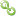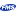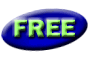Book RecommendationsLinks to Related SitesMy FMS

Thank you! Thank you! I just finished reading this document, which was part of a link in the recent Buzz newsletter. I have printed it for others to read, especially those skeptical on the powers of Access and its capabilities.

Darren D.#Excel Automation: More than just a formula

### Provided by:  FMS Development Team

When you make a call to the Formula property, be aware of the results that are returned. At first, you would expect to either get an empty string or the cell's formula. However, this is not the case.

Here is what could be returned:

• If the cell contains a constant, this property returns the constant.
• If the cell is empty, this formula property returns an empty string.
• If the cell contains a formula, the formula property returns the formula as a string in the same format that would be displayed in the formula bar (including the equal sign).

In your code, to ensure that you are getting a formula returned, you need to check for the "=" character.

Run the sample code below to see the results in the immediate window. Add the code to the click event of a button. Make sure you set the references to a version of Excel.

Here are the results returned in the immediate window:

```The formula property in cell 'A1' says: 10
The formula property in cell 'A4' says: Sum(All Parts)
The formula property in cell 'A6' says: =SUM(A1:A4)
```

## Sample Code

```Dim objExcel As Excel.Application
Dim objActiveSheet As Excel.Worksheet
Dim objWorkbook As Excel.Workbook

Set objExcel = New Excel.Application
Set objWorkbook = objExcel.Workbooks(1)

Set objActiveSheet = objWorkbook.ActiveSheet

objExcel.Visible = True

objActiveSheet.Range("A1").Value = 10
objActiveSheet.Range("A2").Value = 20
objActiveSheet.Range("A3").Value = 30
objActiveSheet.Range("A4").Value = "Sum(All Parts)"

objActiveSheet.Range("A6").Formula = "=Sum(A1:A4)"

' This does NOT display the formula, only the contents of the cell!
Debug.Print "The formula property in cell 'A1' says: " & objActiveSheet.Range("A1").Formula
Debug.Print "The formula property in cell 'A4' says: " & objActiveSheet.Range("A4").Formula

' This DOES display the formula. Notice the EQUAL sign!
Debug.Print "The formula property in cell 'A6' says: " & objActiveSheet.Range("A6").Formula

Set objActiveSheet = Nothing
Set objWorkbook = Nothing
Set objExcel = Nothing
```